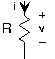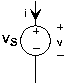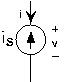# 3.2: Ideal Circuit Elements

Learning Objectives

• This module provides examples of the elementary circuit elements; the resistor, the capacitor,and the inductor, which provide linear relationships between voltage and current.

The elementary circuit elements—the resistor, capacitor, and inductor— impose linear relationships between voltage and current.

## ResistorFig. 3.2.1 Resistor v = Ri

The resistor is far and away the simplest circuit element. In a resistor, the voltage is proportional to the current, with the constant of proportionality R, known as the resistance.

$v(t) = R\, i(t)$

Resistance has units of ohms, denoted by , named for the German electrical scientist Georg Ohm. Sometimes, the v-i relation for the resistor is written i = Gv, with G, the conductance, equal to 1/R. Conductance has units of Siemens (S), and is named for the German electronics industrialist Werner von Siemens.

When resistance is positive, as it is in most cases, a resistor consumes power. A resistor's instantaneous power consumption can be written one of two ways.

$p(t) = R\, i^{2}(t) = \frac{1}{R}v^{2}(t)$

As the resistance approaches infinity, we have what is known as an open circuit: No current flows but a non-zero voltage can appear across the open circuit. As the resistance becomes zero, the voltage goes to zero for a non-zero current flow. This situation corresponds to a short circuit. A superconductor physically realizes a short circuit.

## CapacitorFig. 3.2.2 Capacitor

$i = C\frac{\mathrm{d} v(t)}{\mathrm{d} t}$

The capacitor stores charge and the relationship between the charge stored and the resultant voltage is The constant of proportionality, the capacitance, has units of farads (F), and is named for the English experimental physicist Michael Faraday. As current is the rate of change of charge, the v-i relation can be expressed in differential or integral form.

$i(t) = C\frac{\mathrm{d} v(t)}{\mathrm{d} t}\; or\; v(t) = \frac{1}{C}\int_{-\infty }^{t}i(\alpha )d\alpha$

If the voltage across a capacitor is constant, then the current flowing into it equals zero. In this situation, the capacitor is equivalent to an open circuit. The power consumed/produced by a voltage applied to a capacitor depends on the product of the voltage and its derivative.

$p(t) = Cv(t)\frac{\mathrm{d} v(t)}{\mathrm{d} t}$

This result means that a capacitor's total energy expenditure up to time t is concisely given by

$E(t) = \frac{1}{2}Cv^{2}(t)$

This expression presumes the fundamental assumption of circuit theory: all voltages and currents in any circuit were zero in the far distant past (t = -∞).

## InductorFig. 3.2.3 Inductor

$v = L\frac{\mathrm{d} i(t)}{\mathrm{d} t}$

The inductor stores magnetic flux, with larger valued inductors capable of storing more flux. Inductance has units of henries (H), and is named for the American physicist Joseph Henry. The differential and integral forms of the inductor's v-i relation are

$v(t) = L\frac{\mathrm{d} i(t)}{\mathrm{d} t}\; or\; i(t) = \frac{1}{L}\int_{-\infty }^{t}v(\alpha )d\alpha$

The power consumed/produced by an inductor depends on the product of the inductor current and its derivative

$p(t) = Li(t)\frac{\mathrm{d} i(t)}{\mathrm{d} t}$

and its total energy expenditure up to time $tt$ is given by

$E(t) = \frac{1}{2}Li^{2}(t)$

## SourcesFig. 3.2.4 The voltage source on the left and current source on the right are like all circuit elements in that they have a particular relationship between the voltage and current defined for them. For the voltage source, v=vs for any current for the current source, i=-is for any voltage

Sources of voltage and current are also circuit elements, but they are not linear in the strict sense of linear systems. For example, the voltage source's v-i relation is v=vs regardless of what the current might be. As for the current source, i=-is regardless of the voltage. Another name for a constant-valued voltage source is a battery, and can be purchased in any supermarket. Current sources, on the other hand, are much harder to acquire; we'll learn why later.

## Contributor

• ContribEEOpenStax